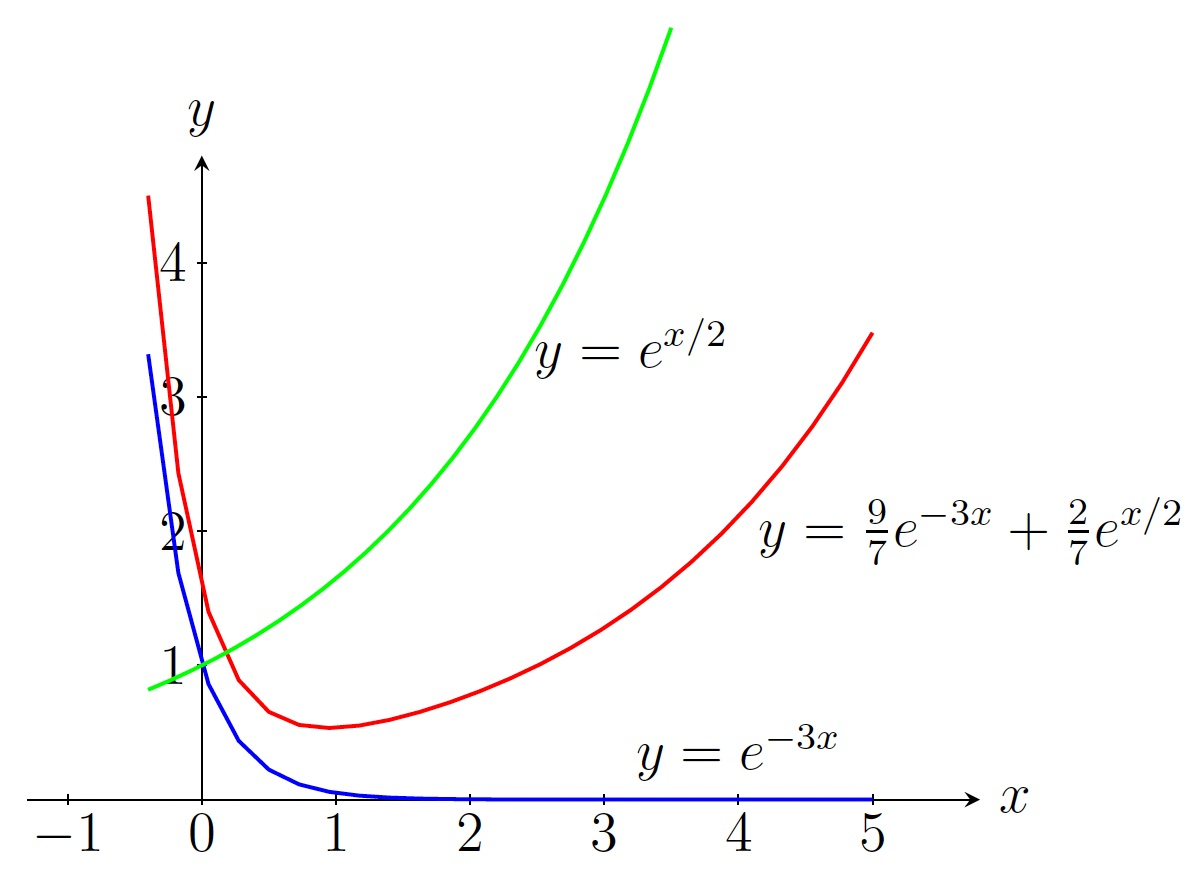DiffEq Home

## Linear Homogeneous ODEs with Constant Coefficients

In this section, we learn how to solve a linear homogeneous second order ODE with constant coefficients. $a\frac{d^2y}{dx^2}+b\frac{dy}{dx}+cy=0, \text{ where } a\neq 0 \;\;\;\;(27)$ Suppose $$y=e^{rx}$$ is a solution of (27) for some values of $$r$$. Then $$y'=re^{rx}$$ and $$y''=r^2e^{rx}$$. Plugging these into (27), we get \begin{align*} ar^2e^{rx}+bre^{rx}+ce^{rx}&=0\\ e^{rx}(ar^2+br+c)&=0 \end{align*} Since $$e^{rx}\neq 0$$, we get a quadratic equation in $$r$$, called the characteristic equation of (27): $ar^2+br+c=0 \;\;\;\;(28)$ There are three possibilities for the roots $$r_1$$ and $$r_2$$ of (28):

1. $$r_1$$ and $$r_2$$ are real and distinct

2. $$r_1$$ and $$r_2$$ are real and the same (see Section 3.3)

3. $$r_1$$ and $$r_2$$ are complex conjugates (see Section 3.4)

For this section assume that $$r_1$$ and $$r_2$$ are real and distinct. Then we have two solutions $$y=e^{r_1x}$$ and $$y=e^{r_2x}$$ for (27). It can be verified that any of their linear combinations would also be a solution of (27) (principle of superposition). Thus we get the general solution: $y=c_1e^{r_1x}+c_2e^{r_2x}$ Now $$c_1$$ and $$c_2$$ can be found using two initial conditions as discussed before.

Steps to solve:

1. Find the characteristic equation $$ar^2+br+c=0$$.

2. Find the (distinct) roots $$r_1$$ and $$r_2$$.

3. The general solution is $$y=c_1e^{r_1x}+c_2e^{r_2x}$$.

4. Find $$c_1$$ and $$c_2$$ when two initial conditions are given.

Example. Find the general solution of the following ODE. $\frac{d^2y}{dx^2}-2\frac{dy}{dx}-3y=0$ Solution. The characteristic equation is \begin{align*} r^2-2r-3&=0\\ (r+1)(r-3)&=0\\ r&=-1,3 \end{align*} So the general solution is $y=c_1e^{-x}+c_2e^{3x}.$
Example. Solve the following IVP. $2y''+5y'-3y=0,\;\; y(0)=11/7,\, y'(0)=-26/7$ Solution. The characteristic equation is \begin{align*} 2r^2+5r-3&=0\\ (2r-1)(r+3)&=0\\ r&=-3,1/2 \end{align*} So the general solution is $y=c_1e^{-3x}+c_2e^{x/2}.$ Using the initial condition $$y(0)=11/7$$, we get $c_1+c_2=11/7. \;\;\;\;(29)$ Note that $$y'=-3c_1e^{-3x}+\frac{c_2}{2}e^{x/2}.$$ Using the initial condition $$y'(0)=-26/7$$, we get $-3c_1+c_2/2=-26/7. \;\;\;\;(30)$ Solving (29) and (30), we get $$c_1=9/7$$ and $$c_2=2/7$$. Thus the solution of the IVP is $y=\frac{9}{7} e^{-3x}+\frac{2}{7} e^{x/2}.$Last edited